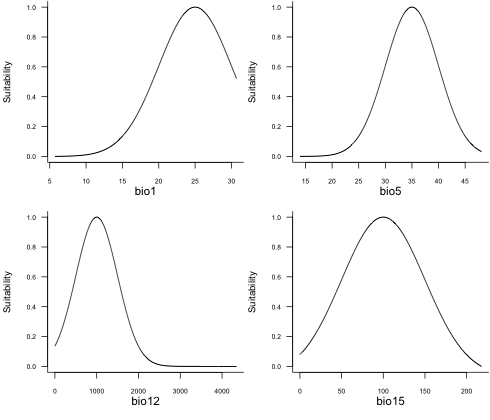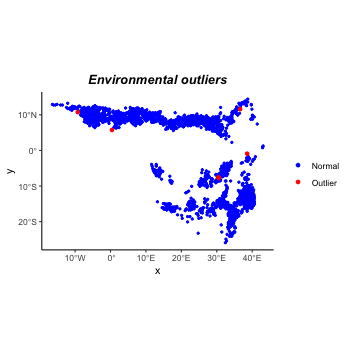## Set up

``````install.packages('rnaturalearth')
install.packages('here')
install.packages('virtualspecies')``````
``````library(sf, quietly = T)
library(itsdm, quietly = T)
library(ggplot2, quietly = T)
library(dplyr, quietly = T)
select <- dplyr::select``````

## Prepare environmental variables

We could use packages like `rnaturalearth` to quickly get the boundary of most countries and regions. You can also read your study area boundary for sure. Providing your boundary to function `worldclim2` would allow you to download files from worldclim version 2 clipping to your area.

``````library(stars, quietly = T)
library(rnaturalearth, quietly = T)

# Get Africa continent
af <- ne_countries(
continent = 'africa', returnclass = 'sf') %>%

# Union countries to continent
af_bry <- st_buffer(af, 0.1) %>%
st_union() %>%
st_as_sf() %>%
rename(geometry = x) %>%
st_make_valid()

bios <- worldclim2(var = 'bio', bry = af_bry,
path = tempdir(),
nm_mark = 'africa')

# Plot BIO1 to check the variables
# plot(bios %>% slice('band', 1),
#      main = st_get_dimension_values(bios, 'band'))``````

In species modeling, people usually want to remove the strong correlations between environmental variables. `dim_reduce` is such a function you need. The function could either reduce the dimension of your environmental variable stack itself or according to a bunch of observations. It also allows you to set a desirable threshold. Note that it only works on numeric variables. Because categorical variables have less risk of having a high correlation with others, we usually prefer to keep categorical variables.

``````library(stars, quietly = T)
# An example of reducing dimensions
## Here we didn't set samples, so use whole image
bios_reduce <- dim_reduce(
bios, threshold = 0.6,
preferred_vars = c('bio1', 'bio12', 'bio5'))

# Returned ReducedImageStack object
bios_reduce
#> Dimension reduction
#> Correlation threshold: 0.6
#> Original variables: bio1, bio2, bio3, bio4, bio5, bio6, bio7, bio8, bio9,
#> bio10, bio11, bio12, bio13, bio14, bio15, bio16, bio17, bio18, bio19
#> Variables after dimension reduction: bio1, bio12, bio9, bio14, bio15
#> ================================================================================
#> Reduced correlations:
#>        bio1 bio12  bio9 bio14 bio15
#> bio1   1.00 -0.04  0.50 -0.07  0.44
#> bio12 -0.04  1.00 -0.03  0.56 -0.16
#> bio9   0.50 -0.03  1.00  0.01 -0.06
#> bio14 -0.07  0.56  0.01  1.00 -0.40
#> bio15  0.44 -0.16 -0.06 -0.40  1.00

# img_reduced of ReducedImageStack is the raster stack
bios_reduce\$img_reduced
#> stars object with 3 dimensions and 1 attribute
#> attribute(s):
#>                Min.  1st Qu.   Median     Mean  3rd Qu. Max.   NA's
#> reduced_image     0 17.75392 25.91388 154.5419 83.09974 4347 447385
#> dimension(s):
#>      from   to offset     delta refsys point         values x/y
#> x     975 1388   -180  0.166667 WGS 84 FALSE           NULL [x]
#> y     316  750     90 -0.166667 WGS 84 FALSE           NULL [y]
#> band    1    5     NA        NA     NA    NA bio1,...,bio15``````

## Creating the virtual species

Using virtual species is a crucial method in ecological studies. First, let’s create a virtual species using the package `virtualspecies` to know exactly what is happening.

``````library(here, quietly = T)
library(virtualspecies, quietly = T)

# Subset environmental variables to use
bios_sub <- bios %>% slice('band', c(1, 5, 12, 15))
bios_sub <- stack(as(split(bios_sub), 'Spatial'))

# Formatting of the response functions
set.seed(10)
my.parameters <- formatFunctions(
bio1 = c(fun = 'dnorm', mean = 25, sd = 5),
bio5 = c(fun = 'dnorm', mean = 35, sd = 5),
bio12 = c(fun = 'dnorm', mean = 1000, sd = 500),
bio15 = c(fun = 'dnorm', mean = 100, sd = 50))

# Generation of the virtual species
set.seed(10)
my.species <- generateSpFromFun(
raster.stack = bios_sub,
parameters = my.parameters,
plot = F)

# Conversion to presence-absence
set.seed(10)
my.species <- convertToPA(
my.species,
beta = 0.7,
plot = F)

# Check maps of this virtual species if you like
# plot(my.species)

# Check response curves
plotResponse(my.species)``````## Generate pseudo samples for virtual species

``````# Sampling
set.seed(10)
po.points <- sampleOccurrences(
my.species,
n = 2000,
type = "presence only",
plot = FALSE)
po_df <- po.points\$sample.points %>%
select(x, y) %>%
mutate(id = row_number())
#>           x          y id
#> 1 -6.083333  11.083333  1
#> 2 -5.750000  10.250000  2
#> 3 38.750000  -6.750000  3
#> 4 39.250000 -10.416667  4
#> 5 26.583333  -8.583333  5
#> 6  0.250000   9.083333  6``````

As we all know, there are commonly sampling bias and observation errors. People use multiple methods to reduce these disturbances in samples. For example, here, we use the function `suspicious_env_outliers` to detect and/or remove possible environmental outliers. This step could be used with other strategies to do sample cleaning.

``````# Get environmental variable stack
variables <- bios %>% slice('band', c(1, 5,  12, 15))

# Check outliers
occ_outliers <- suspicious_env_outliers(
po_df,
variables = variables,
z_outlier = 5,
outliers_print = 4L)
#> Reporting top 4 outliers [out of 6 found]
#>
#> row  - suspicious column: [bio5] - suspicious value: [32.78]
#>  distribution: 95.714% >= 36.01 - [mean: 37.68] - [sd: 0.66] - [norm. obs: 67]
#>  given:
#>      [bio1] > [27.31] (value: 27.39)
#>      [bio15] <= [102.79] (value: 102.48)
#>      [bio12] <= [993.00] (value: 845.00)
#>
#>
#> row  - suspicious column: [bio5] - suspicious value: [32.96]
#>  distribution: 95.714% >= 36.01 - [mean: 37.68] - [sd: 0.66] - [norm. obs: 67]
#>  given:
#>      [bio1] > [27.31] (value: 27.41)
#>      [bio15] <= [102.79] (value: 102.34)
#>      [bio12] <= [993.00] (value: 855.00)
#>
#>
#> row  - suspicious column: [bio5] - suspicious value: [33.42]
#>  distribution: 95.714% >= 36.01 - [mean: 37.68] - [sd: 0.66] - [norm. obs: 67]
#>  given:
#>      [bio1] > [27.31] (value: 27.44)
#>      [bio15] <= [102.79] (value: 66.08)
#>      [bio12] <= [993.00] (value: 958.00)
#>
#>
#> row  - suspicious column: [bio5] - suspicious value: [31.90]
#>  distribution: 98.333% >= 34.51 - [mean: 36.25] - [sd: 0.84] - [norm. obs: 59]
#>  given:
#>      [bio1] between (24.12, 25.70] (value: 24.70)
#>      [bio15] > [113.08] (value: 128.18)
#>      [bio12] <= [996.00] (value: 658.00)

# Check result
# You could also plot samples overlap with a raster
# plot(occ_outliers,
#      overlay_raster = variables %>% slice('band', 6))
plot(occ_outliers)````````````
# Remove outliers if necessary
occ_outliers <- suspicious_env_outliers(
po_df, variables = variables,
rm_outliers = T,
z_outlier = 5,
outliers_print = 0L)
po_sf <- occ_outliers\$pts_occ

# Make occurrences
set.seed(11)
occ_sf <- po_sf %>% sample_frac(0.7)
occ_test_sf <- po_sf %>% filter(! id %in% occ_sf\$id)
occ_sf <- occ_sf %>% select(-id)
occ_test_sf <- occ_test_sf %>% select(-id)

# Have a look at the samples if you like
# ggplot() +
#   geom_raster(data = as.data.frame(my.species\$suitab.raster, xy = T),
#               aes(x, y, fill = layer)) +
#   scale_fill_viridis_c('Suitability', na.value = 'transparent') +
#   geom_sf(data = occ_sf, aes(color = 'Train'), size = 0.8) +
#   geom_sf(data = occ_test_sf, aes(color = 'Test'), size = 0.8) +
#   scale_color_manual('', values = c('Train' = 'red', 'Test' = 'blue')) +
#   theme_classic()

# Recheck the variable correlation
dim_reduce(variables, threshold = 1.0, samples = occ_sf)
#> Dimension reduction
#> Correlation threshold: 1
#> Original variables: bio1, bio5, bio12, bio15
#> Variables after dimension reduction: bio1, bio5, bio12, bio15
#> ================================================================================
#> Reduced correlations:
#>        bio1  bio5 bio12 bio15
#> bio1   1.00  0.69  0.19 -0.22
#> bio5   0.69  1.00 -0.03  0.20
#> bio12  0.19 -0.03  1.00 -0.37
#> bio15 -0.22  0.20 -0.37  1.00``````

Unfortunately, bio1 and bio5 have strong correlation with each other. This might affect the model explanation later.

## Build a simple `isolation_forest` species distribution model

Here we build a SDM using extended isolation forest (with `ndim = 2`) and a sample rate of 0.8.

``````# Do modeling
it_sdm <- isotree_po(occ = occ_sf,
occ_test = occ_test_sf,
variables = variables,
sample_size = 0.8,
ndim = 2)``````

Let’s compare the predicted suitability with real suitability.